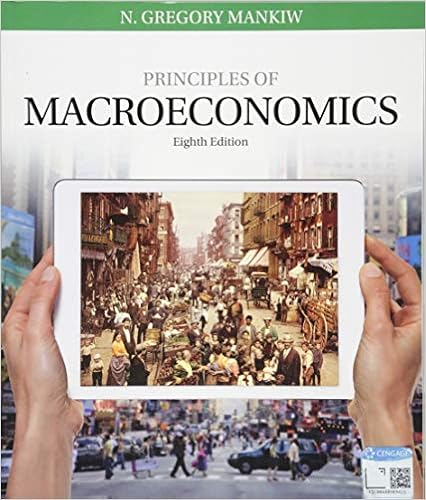# The sign negative or positive on the cross price

• Test Prep
• 5
• 63% (8) 5 out of 8 people found this document helpful

This preview shows page 2 - 4 out of 5 pages.

##### We have textbook solutions for you!
The document you are viewing contains questions related to this textbook.The document you are viewing contains questions related to this textbook.
Chapter 11 / Exercise 3
Principles of Macroeconomics
MankiwExpert Verified
11. The sign (negative or positive) on the cross-price elasticity of demand for wine and chocolate
##### We have textbook solutions for you!
The document you are viewing contains questions related to this textbook.The document you are viewing contains questions related to this textbook.
Chapter 11 / Exercise 3
Principles of Macroeconomics
MankiwExpert Verified
tells us:
12. What do you have to know in order to calculate the price elasticity of supply?
13. The sign (positive or negative) on the income elasticity of demand tells us:
14. If the price elasticity of demand (calculated using the mid-point method) for -eBooks is 2.5, and the price of e-Books rises from \$18 to \$22, then the quantity demanded will: a. decrease by 8%.b. decrease by 25%.c. decrease by 50%.d. increase by 2.5%.
15. The demand for a good becomes relatively more elastic as:
16. What happens to the elasticity of demand as we move down to the right along a straight-line demand curve?
•••